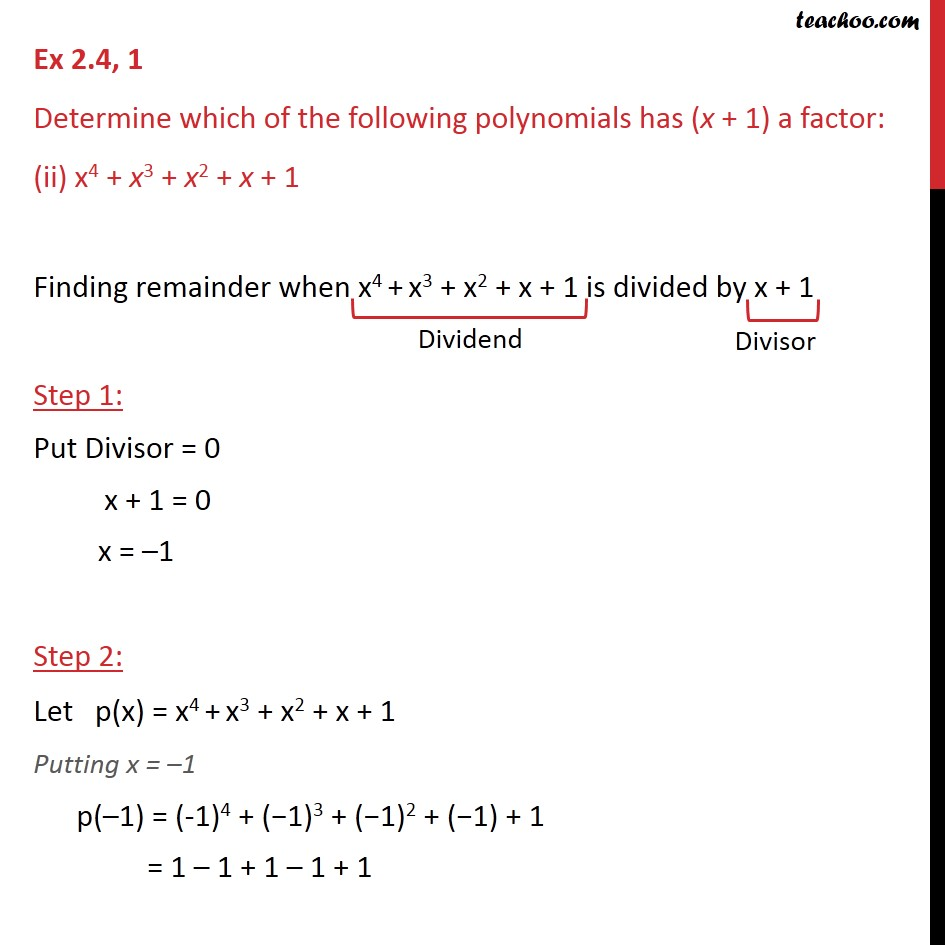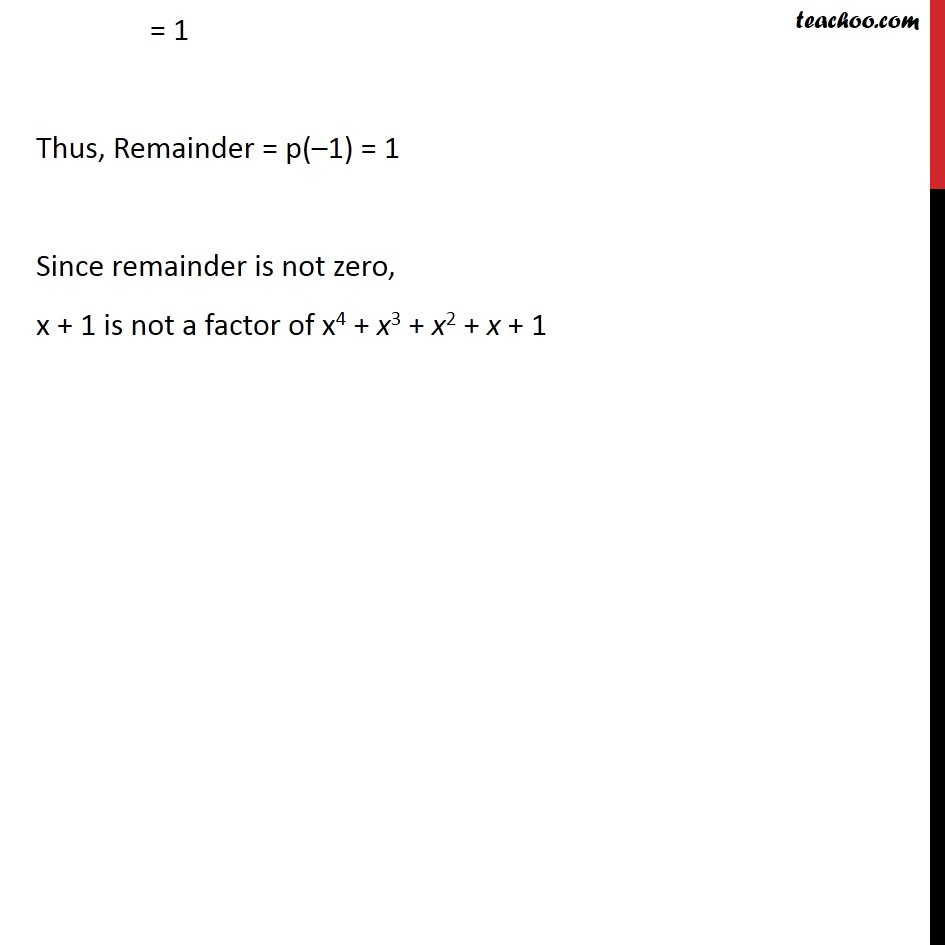Ex 2.4

Chapter 2 Class 9 Polynomials (Term 2)
Serial order wise### Transcript

Ex 2.4, 1 Determine which of the following polynomials has (x + 1) a factor: (ii) x4 + x3 + x2 + x + 1 Finding remainder when x4 + x3 + x2 + x + 1 is divided by x + 1 Step 1: Put Divisor = 0 x + 1 = 0 x = 1 Step 2: Let p(x) = x4 + x3 + x2 + x + 1 Putting x = 1 p( 1) = (-1)4 + ( 1)3 + ( 1)2 + ( 1) + 1 = 1 1 + 1 1 + 1 = 1 Thus, Remainder = p( 1) = 1 Since remainder is not zero, x + 1 is not a factor of x4 + x3 + x2 + x + 1